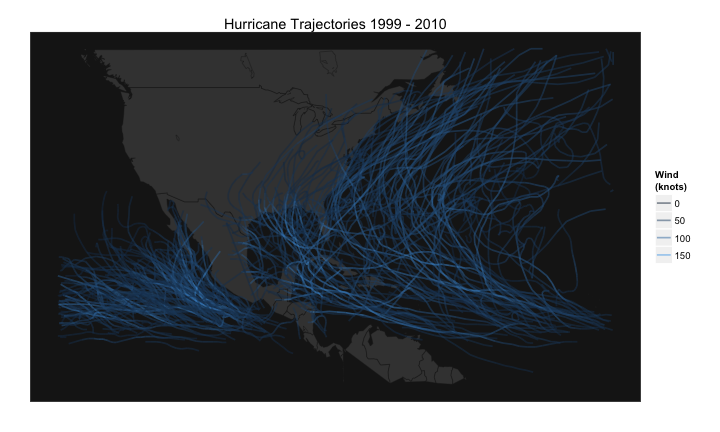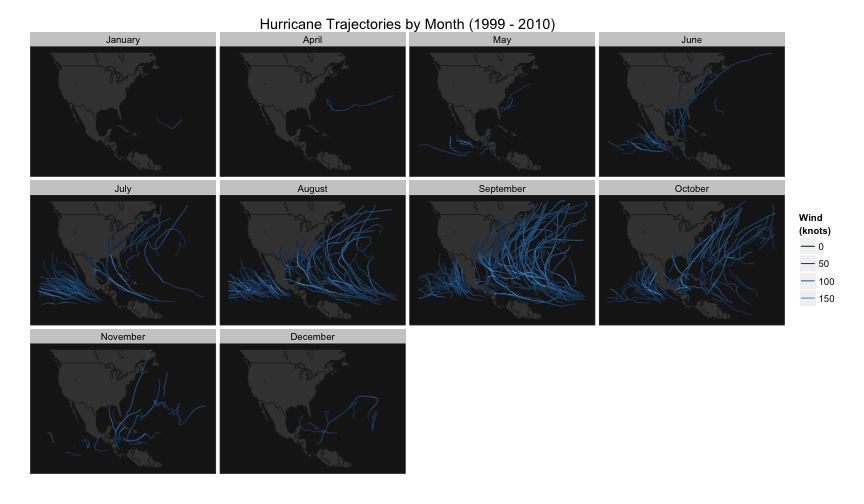# Visualizing Hurricane Trajectories

This RPub shows how to visualize some hurricane trajectories in R.
(Thanks a lot to all the RStudio team for your awesome job!)

### Description

Inspired by Aaron Koblin's flight patterns and by Mapped British Shipping, I decided to play with a different data about storms (i.e. hurricanes) and try to map their trajectories.

### The Data

The data is freely available from the International Best Track Archive for Climate Stewardship (IBTrACS) at the National Climatic Data Center website and can be downloaded in different formats. In my case I decided to use the csv files although you can find a lot of different formats (pick the one that best fits your needs or preferences).

``````# load packages
library(maps)
library(ggplot2)

# NOAA url
noaa = "ftp://eclipse.ncdc.noaa.gov/pub/ibtracs/v03r03/wmo/csv/basin/"

# basins
basin = c("NA", "EP")

NA.basin = read.csv(paste(noaa, "Basin.", basin, ".ibtracs_wmo.v03r03.csv",
sep = ""), skip = 1, stringsAsFactors = FALSE)
EP.basin = read.csv(paste(noaa, "Basin.", basin, ".ibtracs_wmo.v03r03.csv",
sep = ""), skip = 1, stringsAsFactors = FALSE)

# remove variable information
NA.basin = NA.basin[-1, ]
EP.basin = EP.basin[-1, ]

# formatting some columns
NA.basin\$Season = as.numeric(NA.basin\$Season)
NA.basin\$Latitude = as.numeric(gsub("^ ", "", NA.basin\$Latitude))
NA.basin\$Longitude = as.numeric(gsub("^ ", "", NA.basin\$Longitude))
NA.basin\$Wind.WMO. = as.numeric(gsub("^ ", "", NA.basin\$Wind.WMO.))

EP.basin\$Season = as.numeric(EP.basin\$Season)
EP.basin\$Latitude = as.numeric(gsub("^ ", "", EP.basin\$Latitude))
EP.basin\$Longitude = as.numeric(gsub("^ ", "", EP.basin\$Longitude))
EP.basin\$Wind.WMO. = as.numeric(gsub("^ ", "", EP.basin\$Wind.WMO.))

# extract month for dataset NA.basin
time.date = strsplit(NA.basin\$ISO_time, " ")
iso.date = unlist(lapply(time.date, function(x) x))
iso.month = substr(iso.date, 6, 7)
NA.basin\$Month = factor(iso.month, labels = c(month.name))

# extract month for dataset EP.basin
time.date = strsplit(EP.basin\$ISO_time, " ")
iso.date = unlist(lapply(time.date, function(x) x))
iso.month = substr(iso.date, 6, 7)
EP.basin\$Month = factor(iso.month, labels = c(month.name)[-4])

# join data frames
storms = rbind(NA.basin, EP.basin)
``````

### Prepare the plot ingredients

Once we've cleaned and processed the data, the next step is to prepare the ingredients for plotting a map. For this example, I'm selecting hurricanes from 1999 to 2010, and removing unnamed storms:

``````# world map
wm = map_data("world")

# select storms between 1999 and 2010
substorms = subset(storms, Season %in% 1999:2010)
nop = which(substorms\$Name == "NOT NAMED")
substorms = substorms[-nop, ]

# add and ID with name and season
substorms\$ID = as.factor(paste(substorms\$Name, substorms\$Season,
sep = "."))

# storm name as factor
substorms\$Name = as.factor(substorms\$Name)
``````

### Overall Hurricanes

Let's plot the data with all the selected storms

``````# map with ggplot
map1 = ggplot(substorms, aes(x = Longitude, y = Latitude, group = ID)) +
geom_polygon(data = map_data("world"), aes(x = long, y = lat, group = group),
fill = "gray25", colour = "gray10", size = 0.2) + geom_path(data = substorms,
aes(group = ID, colour = Wind.WMO.), alpha = 0.5, size = 0.8) + xlim(-138,
-20) + ylim(3, 55) + labs(x = "", y = "", colour = "Wind \n(knots)") + opts(panel.background = theme_rect(fill = "gray10",
colour = "gray30"), title = "Hurricane Trajectories 1999 - 2010", axis.text.x = theme_blank(),
axis.text.y = theme_blank(), axis.ticks = theme_blank(), panel.grid.major = theme_blank(),
panel.grid.minor = theme_blank())

# show me the map
map1
``````
``````## Warning: Removed 793 rows containing missing values (geom_path).
``````### Hurricanes by month

Let's get a more interesting visualization by months

``````# with facet-wrap by Month
map2 = ggplot(substorms, aes(x = Longitude, y = Latitude, group = ID)) +
geom_polygon(data = map_data("world"), aes(x = long, y = lat, group = group),
fill = "gray25", colour = "gray10", size = 0.2) + geom_path(data = substorms,
aes(group = ID, colour = Wind.WMO.), size = 0.5) + xlim(-138, -20) + ylim(3,
55) + labs(x = "", y = "", colour = "Wind \n(knots)") + facet_wrap(~Month) +
opts(title = "Hurricane Trajectories by Month (1999 - 2010)", panel.background = theme_rect(fill = "gray10",
colour = "gray30"), axis.text.x = theme_blank(), axis.text.y = theme_blank(),
axis.ticks = theme_blank(), panel.grid.major = theme_blank(), panel.grid.minor = theme_blank())

map2
``````
``````## Warning: Removed 3 rows containing missing values (geom_path).
``````
``````## Warning: Removed 21 rows containing missing values (geom_path).
``````
``````## Warning: Removed 86 rows containing missing values (geom_path).
``````
``````## Warning: Removed 356 rows containing missing values (geom_path).
``````
``````## Warning: Removed 145 rows containing missing values (geom_path).
``````
``````## Warning: Removed 85 rows containing missing values (geom_path).
``````
``````## Warning: Removed 9 rows containing missing values (geom_path).
``````Obviously there are many more possibilities to visualize the data but I'll leave them to you. Have fun, and happy data analysis!